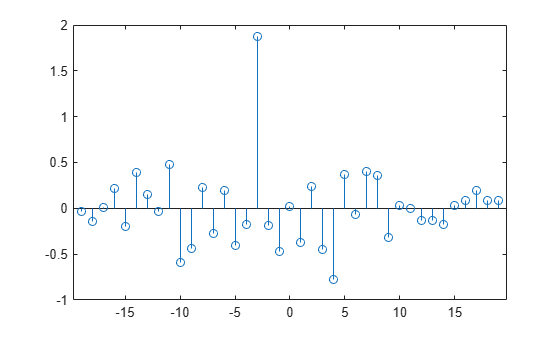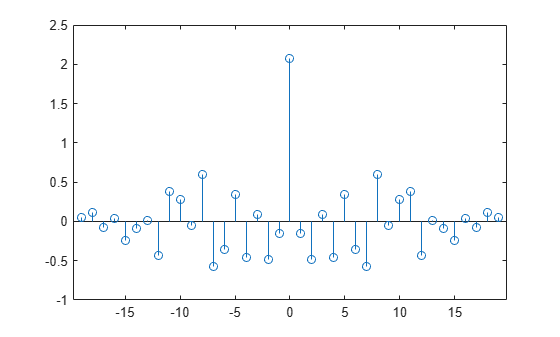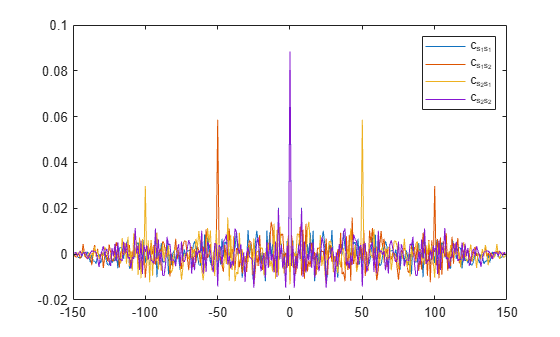Documentation

# xcov

Cross-covariance

## Syntax

``c = xcov(x,y)``
``c = xcov(x)``
``c = xcov(___,maxlag)``
``c = xcov(___,scaleopt)``
``````[c,lags] = xcov(___)``````

## Description

example

````c = xcov(x,y)` returns the cross-covariance of two discrete-time sequences. Cross-covariance measures the similarity between a vector `x` and shifted (lagged) copies of a vector `y` as a function of the lag. If `x` and `y` have different lengths, the function appends zeros to the end of the shorter vector so it has the same length as the other.```

example

````c = xcov(x)` returns the autocovariance sequence of `x`. If `x` is a matrix, then `c` is a matrix whose columns contain the autocovariance and cross-covariance sequences for all combinations of the columns of `x`.```

example

````c = xcov(___,maxlag)` sets the lag range from `-maxlag` to `maxlag` for either of the previous syntaxes.```

example

````c = xcov(___,scaleopt)` also specifies a normalization option for the cross-covariance or autocovariance. Any option other than `'none'` (the default) requires the inputs `x` and `y` to have the same length.```

example

``````[c,lags] = xcov(___)``` also returns the lags at which the covariances are computed.```

## Examples

collapse all

Create a vector of random numbers `x` and a vector `y` that is equal to `x` shifted by 3 elements to the right. Compute and plot the estimated cross-covariance of `x` and `y`. The largest spike occurs at the lag value when the elements of `x` and `y` match exactly (-3).

```rng default x = rand(20,1); y = circshift(x,3); [c,lags] = xcov(x,y); stem(lags,c)```Create a random vector, then compute and plot the estimated autocovariance. The largest spike occurs at zero lag, where the vector is exactly equal to itself.

```rng default x = rand(20,1); [c,lags] = xcov(x); stem(lags,c)```Compute and plot the estimated autocovariance of white Gaussian noise, $c\left(m\right)$, for $-10\le m\le 10$. Normalize the sequence so that it is unity at zero lag.

```rng default x = randn(1000,1); maxlag = 10; [c,lags] = xcov(x,maxlag,'normalized'); stem(lags,c)```Create a signal made up of two signals that are circularly shifted from each other by 50 samples.

```rng default shft = 50; s1 = rand(150,1); s2 = circshift(s1,[shft 0]); x = [s1 s2];```

Compute and plot biased estimates of the autocovariance and mutual cross-covariance sequences. The output matrix `c` is organized as four column vectors such that $c=\left(\begin{array}{cccc}{c}_{{s}_{1}{s}_{1}}& {c}_{{s}_{1}{s}_{2}}& {c}_{{s}_{2}{s}_{1}}& {c}_{{s}_{2}{s}_{2}}\end{array}\right)$. ${\mathit{c}}_{{\mathit{s}}_{1}{\mathit{s}}_{2}}$ has maxima at -50 and +100 and ${\mathit{c}}_{{\mathit{s}}_{2}{\mathit{s}}_{1}}$ has maxima at +50 and -100 as a result of the circular shift.

```[c,lags] = xcov(x,'biased'); plot(lags,c) legend('c_{s_1s_1}','c_{s_1s_2}','c_{s_2s_1}','c_{s_2s_2}')```## Input Arguments

collapse all

Input array, specified as a vector, matrix, or multidimensional array. If `x` is a multidimensional array, then `xcov` operates column-wise across all dimensions and returns each autocovariance and cross-covariance as the columns of a matrix.

Data Types: `single` | `double`
Complex Number Support: Yes

Input array, specified as a vector.

Data Types: `single` | `double`
Complex Number Support: Yes

Maximum lag, specified as an integer scalar. If you specify `maxlag`, the returned cross-covariance sequence ranges from `-maxlag` to `maxlag`. By default, the lag range equals 2N – 1, where N is the greater of the lengths of inputs `x` and `y`.

Data Types: `single` | `double`

Normalization option, specified as one of the following.

• `'none'` — Raw, unscaled cross-covariance. `'none'` is the only valid option when inputs `x` and `y` have different lengths.

• `'biased'` — Biased estimate of the cross-covariance.

• `'unbiased'` — Unbiased estimate of the cross-covariance.

• `'normalized'` or `'coeff'` — Normalizes the sequence so that the autocovariances at zero lag equal 1.

## Output Arguments

collapse all

Cross-covariance or autocovariance, returned as a vector or matrix.

If `x` is an M × N matrix, then `xcov(x)` returns a (2M – 1) × N2 matrix with the autocovariances and cross-covariances of the columns of `x`. If you specify a maximum lag `maxlag`, then the output `c` has size (2 × `maxlag` + 1) × N2.

For example, if `S` has three columns, $\text{S}=\left(\begin{array}{ccc}{x}_{1}& {x}_{2}& {x}_{3}\end{array}\right)$, then the result of `C = xcov(S)` is organized as

`$\text{c}=\left(\begin{array}{lllllllll}{c}_{{x}_{1}{x}_{1}}\hfill & {c}_{{x}_{1}{x}_{2}}\hfill & {c}_{{x}_{1}{x}_{3}}\hfill & {c}_{{x}_{2}{x}_{1}}\hfill & {c}_{{x}_{2}{x}_{2}}\hfill & {c}_{{x}_{2}{x}_{3}}\hfill & {c}_{{x}_{3}{x}_{1}}\hfill & {c}_{{x}_{3}{x}_{2}}\hfill & {c}_{{x}_{3}{x}_{3}}\hfill \end{array}\right).$`

Lag indices, returned as a vector.

collapse all

### Cross-Covariance and Autocovariance

`xcov` computes the mean of its inputs, subtracts the mean, and then calls `xcorr`.

The result of `xcov` can be interpreted as an estimate of the covariance between two random sequences or as the deterministic covariance between two deterministic signals.

The true cross-covariance sequence of two jointly stationary random processes, xn and yn, is the cross-correlation of mean-removed sequences,

`${\varphi }_{xy}\left(m\right)=E\left\{\left({x}_{n+m}-{\mu }_{x}\right){\left({y}_{n}-{\mu }_{y}\right)}^{\ast }\right)\right\},$`

where μx and μy are the mean values of the two stationary random processes, the asterisk denotes complex conjugation, and E is the expected value operator. `xcov` can only estimate the sequence because, in practice, only a finite segment of one realization of the infinite-length random process is available.

By default, `xcov` computes raw covariances with no normalization:

`${c}_{xy}\left(m\right)=\left\{\begin{array}{ll}\sum _{n=0}^{N-m-1}\left({x}_{n+m}-\frac{1}{N}\sum _{i=0}^{N-1}{x}_{i}\right)\left({y}_{n}^{\ast }-\frac{1}{N}\sum _{i=0}^{N-1}{y}_{i}^{\ast }\right),\hfill & m\ge 0,\hfill \\ {c}_{yx}^{\ast }\left(-m\right),\hfill & m<0.\hfill \end{array}$`

The output vector `c` has elements given by

`$\text{c(m)}={c}_{xy}\left(m-N\right),\text{ }m=1,\dots ,2N-1.$`

The covariance function requires normalization to estimate the function properly. You can control the normalization of the correlation by using the input argument `scaleopt`.

 Orfanidis, Sophocles J. Optimum Signal Processing: An Introduction. 2nd Edition. New York: McGraw-Hill, 1996.